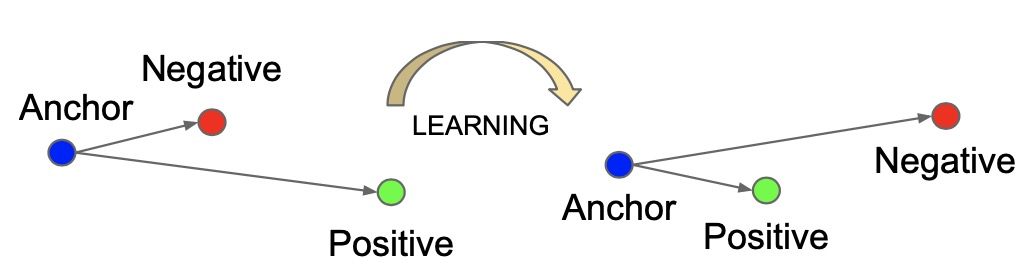# Facenet: A Unified Embedding for Face Recognition and Clustering

## 主要创新点

• FaceNet 将 face verification(判断是否是同一个人)，recognition(判断是何人)和clustering(寻找相似人脸) 任务统一。
• 使用triplet loss 损失函数.

## 方法

• 通过深度模型，将人脸映射为向量(文章设置为128维)，即人脸的 embedding.
• 不同图像之间的相似度通过两个向量的欧几里德距离来决定，距离小则相似度高，距离大则相似度低
• 当得到 embedding vector后：face verification 通过判断两个vector的距离；recognition 成为 k-nn 分类问题；clustering 则通过对vector k-means或其它方法聚类即可.

## 模型结构• 输入一批图像，通过cnn网络提取特征
• 对得到的特征进行 L2正则，得到图像的embedding向量(文章设为128维).
• 根据得到的 embedding 向量计算 triplet loss.## Triplet Loss 的定义

• triplet loss 通过三个参数来计算损失，不在直接算gt 与预测值的 loss.
• anchor, negative, positive 各自对应的 embedding vector 构成三元组.
• anchor 指基准图片, positive 指与anchor 属于同一人的其它任一照片, negative 指与anchor 不属于同一人的任一照片.triplet loss 的意图是希望anchor image（特定人A的图像）与 negative images（所有非人A的图像）相比更接近 positive images（人A的所有图像). 即希望 anchor image 的 embedding vector 与 positive images 的 embedding vector 的距离比 negative images 的距离小(相似度高). 这也是triplet loss 的学习目标.

loss 公式化如下:

$$\sum ^N _i [||f(x ^a _i) - f(x ^p _i)|| ^2 _2 - ||f(x ^a _i) - f(x ^n _i)|| ^2 _2 + \alpha] _ +$$

• $x_i$ 表示一张图像
• $f(x_i)$ 代表图像的 embedding vector
• $\alpha$ 代表 positive-negative pairs 的边际

$$||f(x ^a _i) - f(x ^p _i)|| ^2 _2 + \alpha < ||f(x ^a _i) - f(x ^n _i)|| ^2 _2$$

## Triplet Selection

triplet 的选择对模型的收敛很重要. 为了确保快速收敛，选择违反三元组约束的三元组至关重要.

$$Argmin || f(x ^a _i) - f(x ^n _i)|| ^2 _2, eq2$$

$eq1$ 给定一个人A的anchor image, 想找到一张A的图片positive，以使这两个图像之间的距离最大

$eq2$ 给定一个人A的anchor image, 想找到一张非A的图片negative，以使这两个图像之间的距离最小

• 每 $n$ 步离线生成 triplets, 使用最新的checkpoint并在数据子集上计算argmin和argmax
• 在线生成. 从 mini-batch 中选择 hard positive/ negative 样本.

• 对训练数据进行采样，使mini-batch中的出现的每个身份具有大约40张面孔. 另外，将随机采样的 negative 样本 添加到每个mini-batch中
• 相对于选择 hardest positive, 在mini-batch 中选择所有 anchor-positive 对, 同时选择 hard negative
• 文中认为选择 hardest negatives 会导致 bad 局部极值, 导致无法训练. 作者选择 $semi-hard$, 即: $||f(x ^a _i) - f(x ^p _i)|| ^2 _2 < ||f(x ^a _i) - f(x ^n _i)|| ^2 _2$ , 它们比 positive 样本离 anchor 更远，但是平方距离接近于 anchor-positive距离. 这些 negatives 样本位于边距 $\alpha$内.